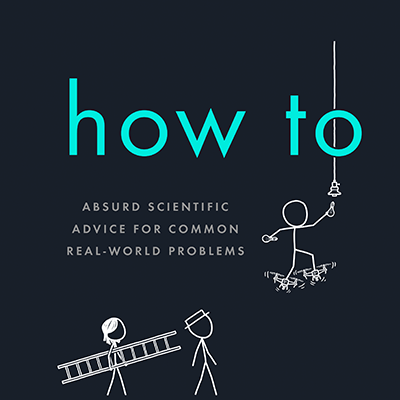serious answers to absurd questions and absurd advice for common concerns from xkcd's Randall Munroe

#### the news

What If? 2: Additional Serious Scientific Answers to Absurd Hypothetical Questions is out! Order here!## Droppings

If you went outside and lay down on your back with your mouth open, how long would you have to wait until a bird pooped in it?

$\text{Period}=\frac{1}{\text{Frequency}}=\frac{1}{\frac{300 \text{ billion birds}}{4\pi\times\text{Earth radius}^2}\times 1\frac{\frac{\text{poop}}{\text{bird}}}{\text{hour}}\times16\frac{\text{hours}}{\text{day}}\times 1 \frac{\text{mouth}}{\text{poop}}\times 15 \frac{\text{ cm}^2}{\text{mouth}}}=195\text{ years}$

Unit cancellation is weird.

Of course, that equation makes a few simplifying assumptions.

It assumes there are 300 billion birds in the world. This number comes from the best-titled source ever—“How many birds are there?”, an actual academic paper published in 1996 in the journal Biodiversity and Conservation.

The equation also assumes birds are randomly distributed across the Earth’s surface (they’re not) and that they poop an average of once an hour at a random location (they don't, although having owned a bird, I can tell you that’s probably more accurate than you might think). In the real world, the time would vary tremendously. For example, if the spot you pick is in a park under a tree, it could turn out to be a matter of hours.However long it takes, though, one thing is certain: Given the relative areas of your mouth and your body, by the time they do finally get your mouth, the rest of you will have been hit an average of several hundred times.

I have to say—from a dimensional analysis standpoint, ”poops” is one of the strangest units I’ve ever tried to cancel in an equation. But there’s another case of odd unit cancellation, common in everyday life, which is—in a way—even weirder: Gas mileage.

In the US, we measure fuel economy in miles/gallon—which could just as easily be written as gallons/mile. (This reciprocal form has some advantages. It’s popular in Europe, where it’s expressed as liters per 100 kilometers.)

But regardless of which units you use, there’s something strange going on here. Miles are units of length, and gallons are volume—which is $$\text{length}^3$$. So gallons/mile is $$\frac{\text{length}^3}{\text{length}}$$. That’s just $$\text{length}^2$$.

Gas mileage is measured in square meters.

You can even plug it into Wolfram|Alpha, and it’ll tell you that 20 MPG is about 0.1 square millimeters (roughly the area of two pixels on a computer screen).

Unit cancellation is weird.

Ok, so what’s the physical interpretation of that number? Is there one?

It turns out there is! If you took all the gas you burned on a trip and stretched it out into a thin tube along your route, 0.1 square millimeters would be the cross-sectional area of that tube.In conclusion:

If the average bird produces half a fluid ounce of poop a day, and Americans drive about 3 trillion miles a year, then in order to satisfy US demand, cars that ran on bird poop would need to get a minimum of:

$\frac{300\text{ billion birds} \times 0.5\frac{\text{fluid oz}}{\text{day}}}{3\text{ trillion}\frac{\mathrm{miles}}{\text{year}}}=0.335 \mathrm{ mm}^2 = 13\text{ miles per gallon}$

Unit cancellation is weird.

#### the books##### What If?

Serious Scientific Answers to Absurd Hypothetical Questions##### Thing Explainer

Complicated Stuff in Simple Words##### How To

Absurd Scientific Advice for Common Real-World Problems